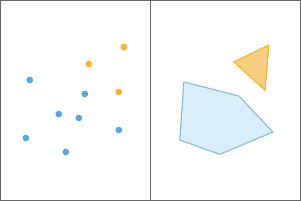# Aggregate functions

Aggregate functions summarize and find relationships between geometries in grouped data. You can group the rows of a DataFrame using one more more column values by calling groupBy on the DataFrame. For example, in this tutorial you will be grouping wind turbines by the name of the project they belong to. By grouping on a string column containing the project name, you can obtain a group of wind turbines for each project.

`DataFrame.groupBy` returns an instance of pyspark.sql.GroupedData, which can be used to calculate the count, max, min, mean, and sum of each column in each group of rows. You can also use it to run any of the aggregate functions in GeoAnalytics Engine.

In this tutorial you will learn how to use ST_Aggr_ConvexHull to calculate the convex hulls of groups of geometries and summarize each group.Two aggregated groups of points (blue and orange) and the resulting convex hulls.

## Prerequisites

The following are required for this tutorial:

1. A running Spark session configured with ArcGIS GeoAnalytics Engine.
2. A notebook connected to your Spark session (e.g. Jupyter, JupyterLab, Databricks, EMR, etc.).
3. An internet connection (for accessing sample data).

## Steps

### Import and authorize

1. In your notebook, import `geoanalytics` and authorize the module using a username and password, or a license file.

Python
Use dark colors for code blocksCopy
``````import geoanalytics
from geoanalytics.sql import functions as ST
from pyspark.sql import functions as F

``````

### Read the sample data and plot

1. Create a DataFrame from a feature service of wind turbine point locations in the United States and print the schema. Apply a filter to only obtain turbines located in the state of Iowa.

Python
Use dark colors for code blocksCopy
``````url = "https://services.arcgis.com/P3ePLMYs2RVChkJx/ArcGIS/rest/services/US_Wind_Turbine_Database/FeatureServer/0"
wind_turbines.printSchema()
``````
Result
Use dark colors for code blocksCopy
``````root
|-- FID: long (nullable = false)
|-- case_id: double (nullable = true)
|-- faa_ors: string (nullable = true)
|-- faa_asn: string (nullable = true)
|-- usgs_pr_id: double (nullable = true)
|-- eia_id: double (nullable = true)
|-- t_state: string (nullable = true)
|-- t_county: string (nullable = true)
|-- t_fips: string (nullable = true)
|-- p_name: string (nullable = true)
|-- p_year: double (nullable = true)
|-- p_tnum: double (nullable = true)
|-- p_cap: double (nullable = true)
|-- t_manu: string (nullable = true)
|-- t_model: string (nullable = true)
|-- t_cap: double (nullable = true)
|-- t_hh: double (nullable = true)
|-- t_rd: double (nullable = true)
|-- t_rsa: double (nullable = true)
|-- t_ttlh: double (nullable = true)
|-- retrofit: double (nullable = true)
|-- retrofit_y: double (nullable = true)
|-- t_conf_atr: double (nullable = true)
|-- t_conf_loc: double (nullable = true)
|-- t_img_date: timestamp (nullable = true)
|-- t_img_srce: string (nullable = true)
|-- xlong: double (nullable = true)
|-- ylat: double (nullable = true)
|-- shape: point (nullable = true)``````
2. Plot the turbine points with a polygon representing the Iowa boundary.

Python
Use dark colors for code blocksCopy
``````axes = wind_turbines.st.plot(figsize=(14, 14))
axes.set(frame_on=False, xticks=[], yticks=[])

url = "https://services.arcgis.com/P3ePLMYs2RVChkJx/ArcGIS/rest/services/USA_States_Generalized/FeatureServer/0"
ia.st.plot(ax=axes, facecolor="none", edgecolor="grey", basemap="light");
``````### Group the data and create convex hulls

1. Group the wind turbines on the `p_name` field which contains the name of the project each turbine belongs to. Then use GroupedData.agg to call `aggr_convex_hull`. The result is a DataFrame with two columns: a polygon column containing the convex hull around each group, and a string column containing the `p_name` of each group.

Python
Use dark colors for code blocksCopy
``````convex_hulls = wind_turbines.groupBy("p_name") \
.agg(ST.aggr_convex_hull("shape").alias("convex_hull"))
convex_hulls.printSchema()
``````
Result
Use dark colors for code blocksCopy
``````root
|-- p_name: string (nullable = true)
|-- convex_hull: polygon (nullable = true)``````
2. Plot the convex hulls with a polygon representing the Iowa boundary.

Python
Use dark colors for code blocksCopy
``````axes = convex_hulls.st.plot(figsize=(14, 14))
axes.set(title="Iowa Wind Power Projects", frame_on=False, xticks=[], yticks=[])

ia.st.plot(ax=axes, facecolor="none", edgecolor="grey", basemap="light");
``````### Group the data and create convex hulls with summary statistics

1. Because GroupedData.agg supports running multiple expressions at once, you can calculate summary statistics for each group in the same function call that creates convex hulls. This can be useful for visualizing the differences between groups or for enriching the result for further analysis.

Perform the same grouping as earlier, except this time calculate the total capacity, minimum year built, maximum height, and average height for each group of wind turbines in addition to the convex hull.

Python
Use dark colors for code blocksCopy
``````convex_hulls_stats = wind_turbines.groupBy("p_name").agg(ST.aggr_convex_hull("shape").alias("convex_hull"),
F.sum("t_cap").alias("sum_capacity"),
F.min("p_year").alias("min_year_built"),
F.max("t_ttlh").alias("max_height"),
F.avg("t_ttlh").alias("avg_height"),
F.count("p_name").alias("count"))
convex_hulls_stats.printSchema()
``````
Result
Use dark colors for code blocksCopy
``````root
|-- p_name: string (nullable = true)
|-- convex_hull: polygon (nullable = true)
|-- sum_capacity: double (nullable = true)
|-- min_year_built: double (nullable = true)
|-- max_height: double (nullable = true)
|-- avg_height: double (nullable = true)
|-- count: long (nullable = false)``````
2. Plot the convex hulls and symbolize on the total capacity of each project.

Python
Use dark colors for code blocksCopy
``````axes = convex_hulls_stats.st.plot(cmap_values="sum_capacity", vmin=2000, figsize=(14, 14), legend=True,
legend_kwds={"label": "Total Capacity (W)", "orientation": "horizontal",
"location": "bottom", "shrink": 0.8, "pad": 0.01})
axes.set(title="Iowa Wind Power Projects", frame_on=False, xticks=[], yticks=[])

ia.st.plot(ax=axes, facecolor="none", edgecolor="grey", basemap="light");``````## What's next?

While this tutorial only shows examples of ST_Aggr_ConvexHull, the same concepts apply to each aggregate function, including: